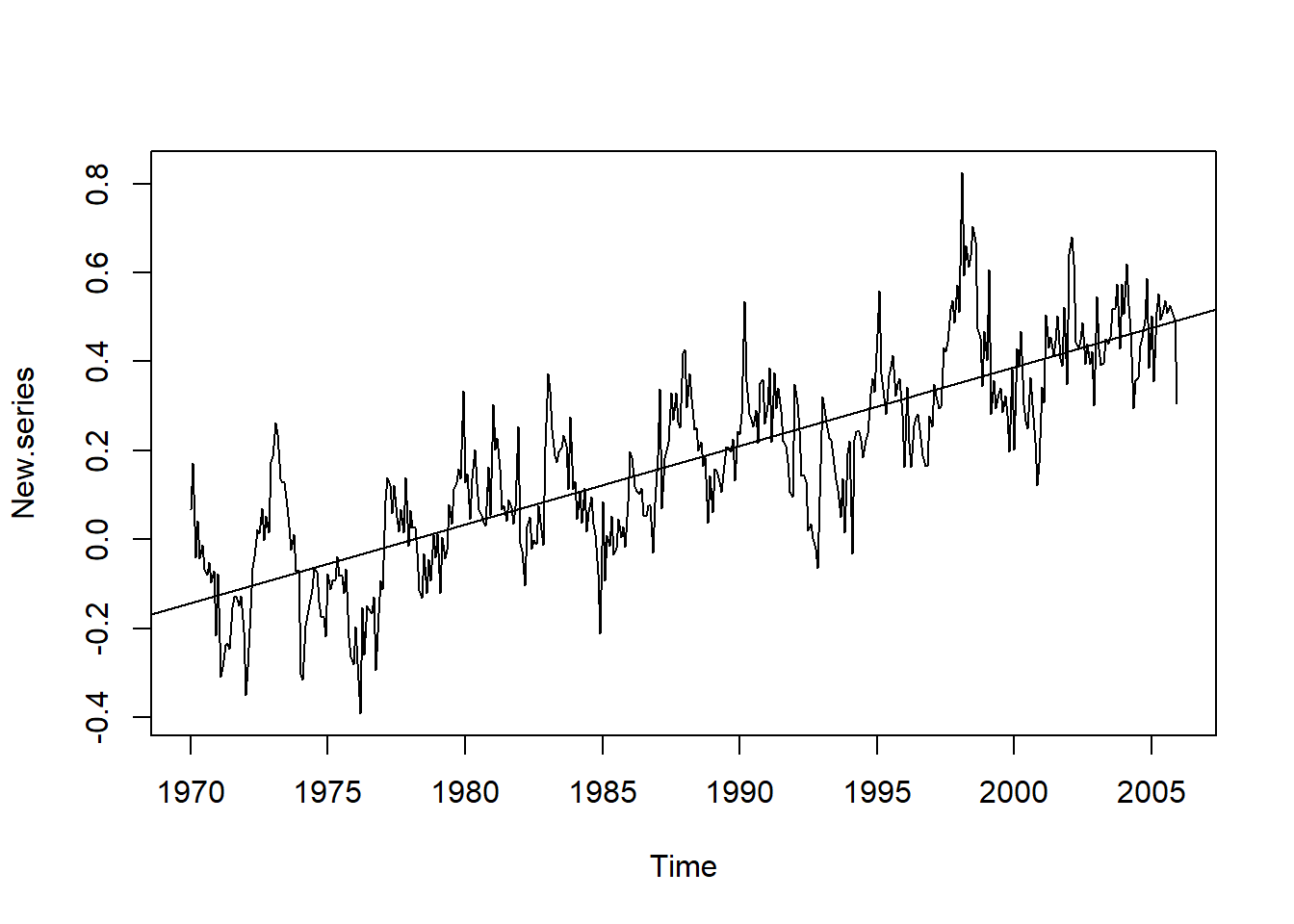# Simple Time Series

## AirPassengers Data

Lets Look at an existing time series

data("AirPassengers")
AP <- AirPassengers
AP
##      Jan Feb Mar Apr May Jun Jul Aug Sep Oct Nov Dec
## 1949 112 118 132 129 121 135 148 148 136 119 104 118
## 1950 115 126 141 135 125 149 170 170 158 133 114 140
## 1951 145 150 178 163 172 178 199 199 184 162 146 166
## 1952 171 180 193 181 183 218 230 242 209 191 172 194
## 1953 196 196 236 235 229 243 264 272 237 211 180 201
## 1954 204 188 235 227 234 264 302 293 259 229 203 229
## 1955 242 233 267 269 270 315 364 347 312 274 237 278
## 1956 284 277 317 313 318 374 413 405 355 306 271 306
## 1957 315 301 356 348 355 422 465 467 404 347 305 336
## 1958 340 318 362 348 363 435 491 505 404 359 310 337
## 1959 360 342 406 396 420 472 548 559 463 407 362 405
## 1960 417 391 419 461 472 535 622 606 508 461 390 432

All data in R are stored in objects, which have a range of methods available. The class of an object can be found using the class function:

class(AP)
##  "ts"

Thus AP is the object of the class ts

start(AP);end(AP);frequency(AP)
##  1949    1
##  1960   12
##  12

start(), end() and frequency() are the methods of the AP object of ts class. Lets see the summary of AP

summary(AP)
##    Min. 1st Qu.  Median    Mean 3rd Qu.    Max.
##   104.0   180.0   265.5   280.3   360.5   622.0

### Plotting a Time Series

Lets plot the time series

plot(AP,ylab="Passengers(1000's)")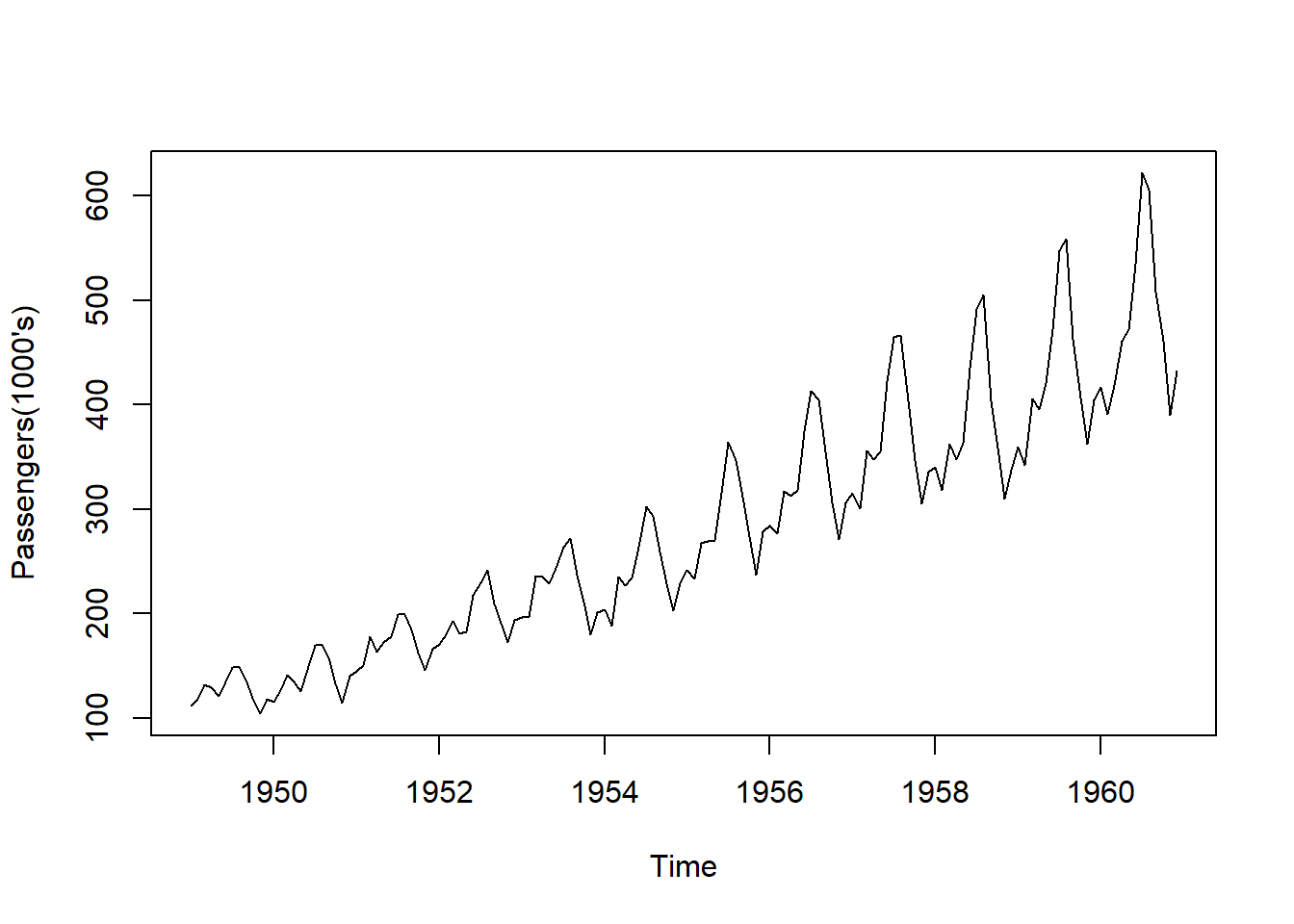### Aggregate Function- Removing the Seasonal Effect

The seasonal effect can be removed by using the “aggregate” function. A summary of the values for each season can be viewed using a boxplot. Cycle function being used to extract the seasons for each items of data. The Plots can be put in a single graphic window using the layout function

layout(1:2)
plot(aggregate(AP))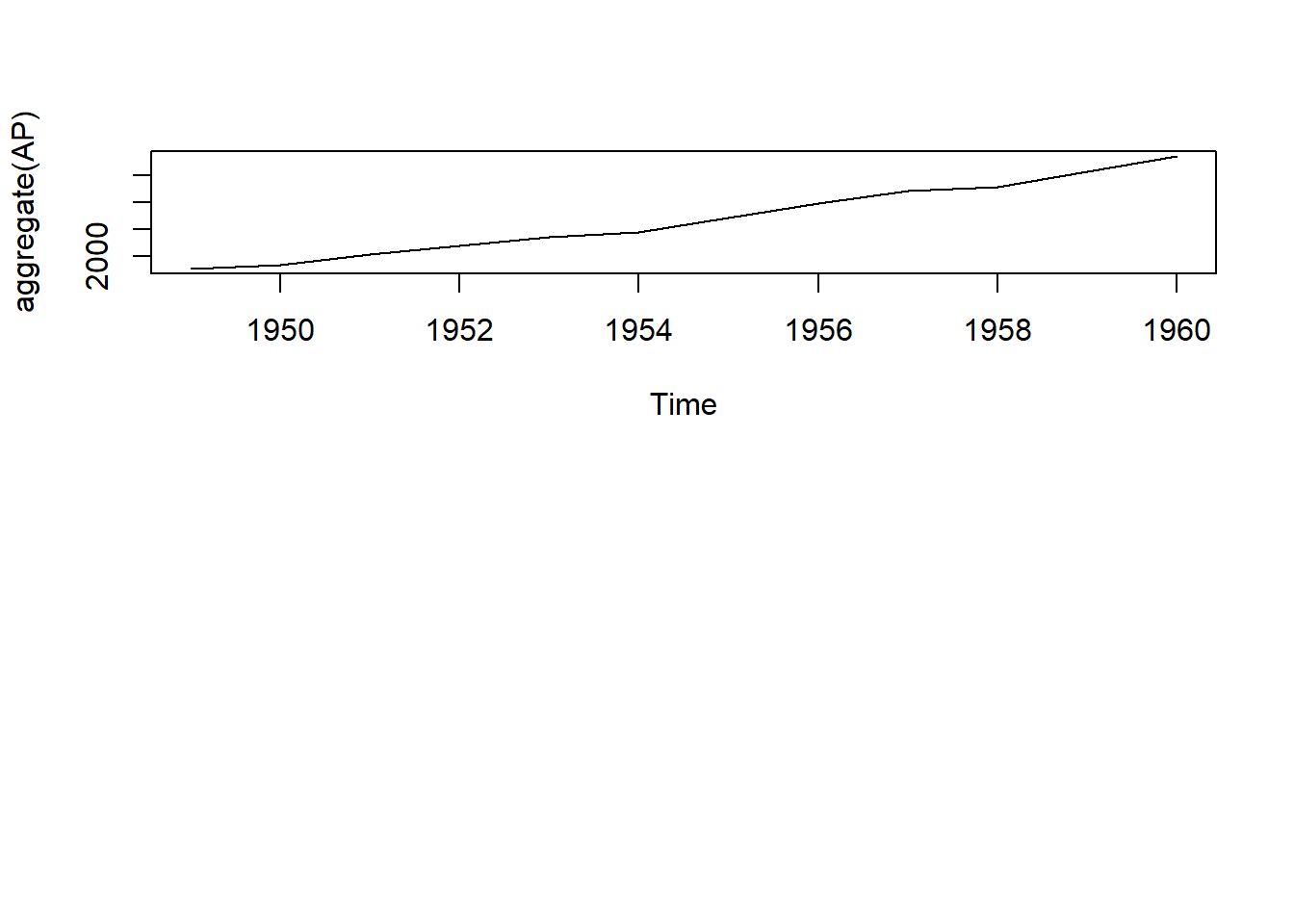boxplot(AP~cycle(AP))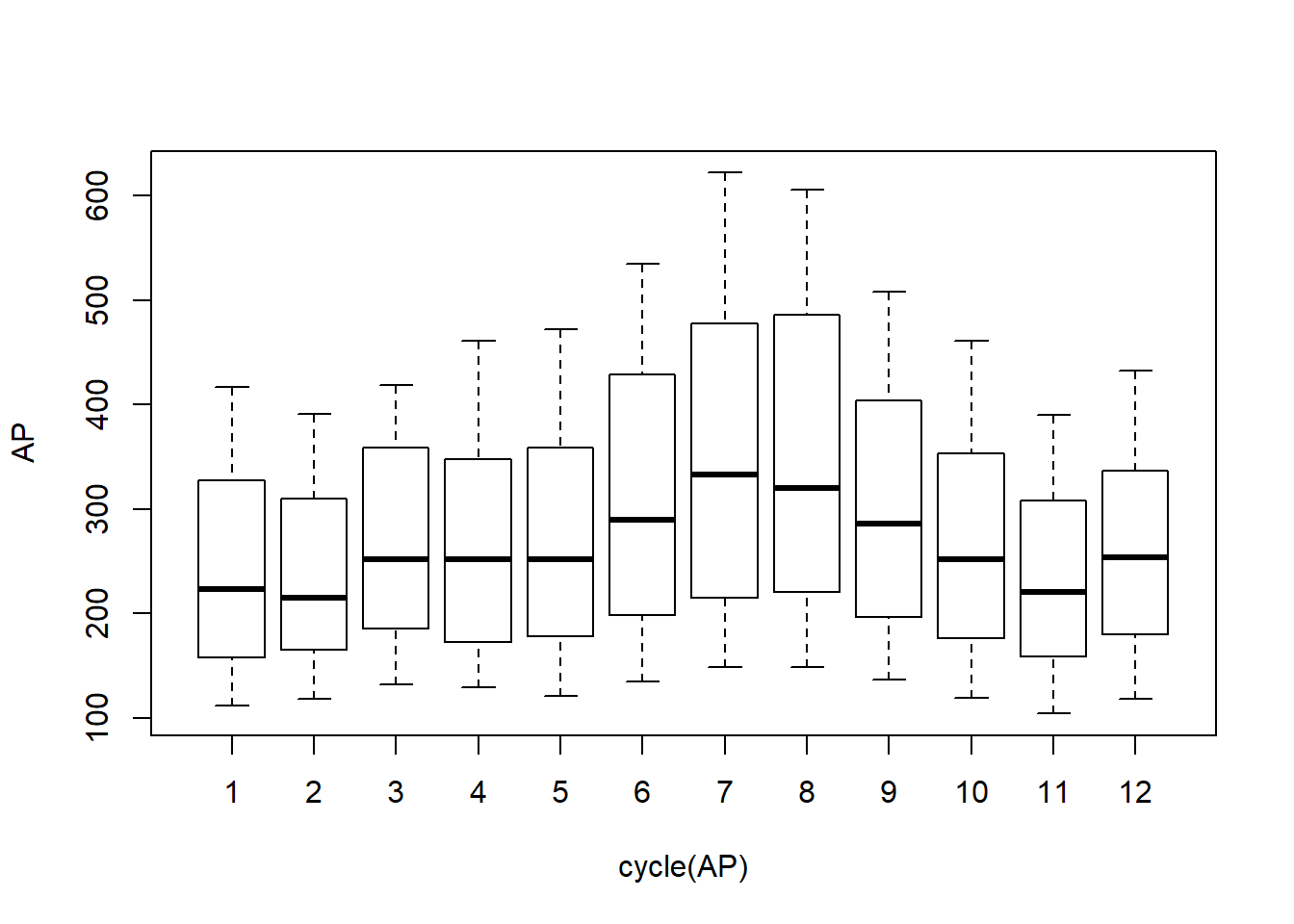## Maine Dataset

Maine.month <- read.table("ts/Maine.dat",header=TRUE)
head(Maine.month)
##   unemploy
## 1      6.7
## 2      6.7
## 3      6.4
## 4      5.9
## 5      5.2
## 6      4.8

So it has one variable “unemploy”

class(Maine.month);str(Maine.month)
##  "data.frame"
## 'data.frame':    128 obs. of  1 variable:
##  $unemploy: num 6.7 6.7 6.4 5.9 5.2 4.8 4.8 4 4.2 4.4 ... ### Converting a data frame to ts object. ts function is used to convert a data to a time series object  Maine.month.ts <- ts(Maine.month$unemploy, start = c(1996, 1), freq = 12)

Looking at the structure and class

str(Maine.month.ts)
##  Time-Series [1:128] from 1996 to 2007: 6.7 6.7 6.4 5.9 5.2 4.8 4.8 4 4.2 4.4 ...

The following code gives the average employment rate over every month. First we aggregated every year, then divide by 12 to get the average of every month.

Maine.annual.ts <- aggregate(Maine.month.ts)/12
Maine.annual.ts
## Time Series:
## Start = 1996
## End = 2005
## Frequency = 1
##   5.258333 5.125000 4.508333 3.950000 3.275000 3.733333 4.341667
##   4.991667 4.616667 4.841667

Lets plot both together

layout(1:2)
plot(Maine.month.ts,ylab="Unemployed(%)",main="Maine.Month")
plot(Maine.annual.ts,ylab="unemployed(%)",main="Maine Annual")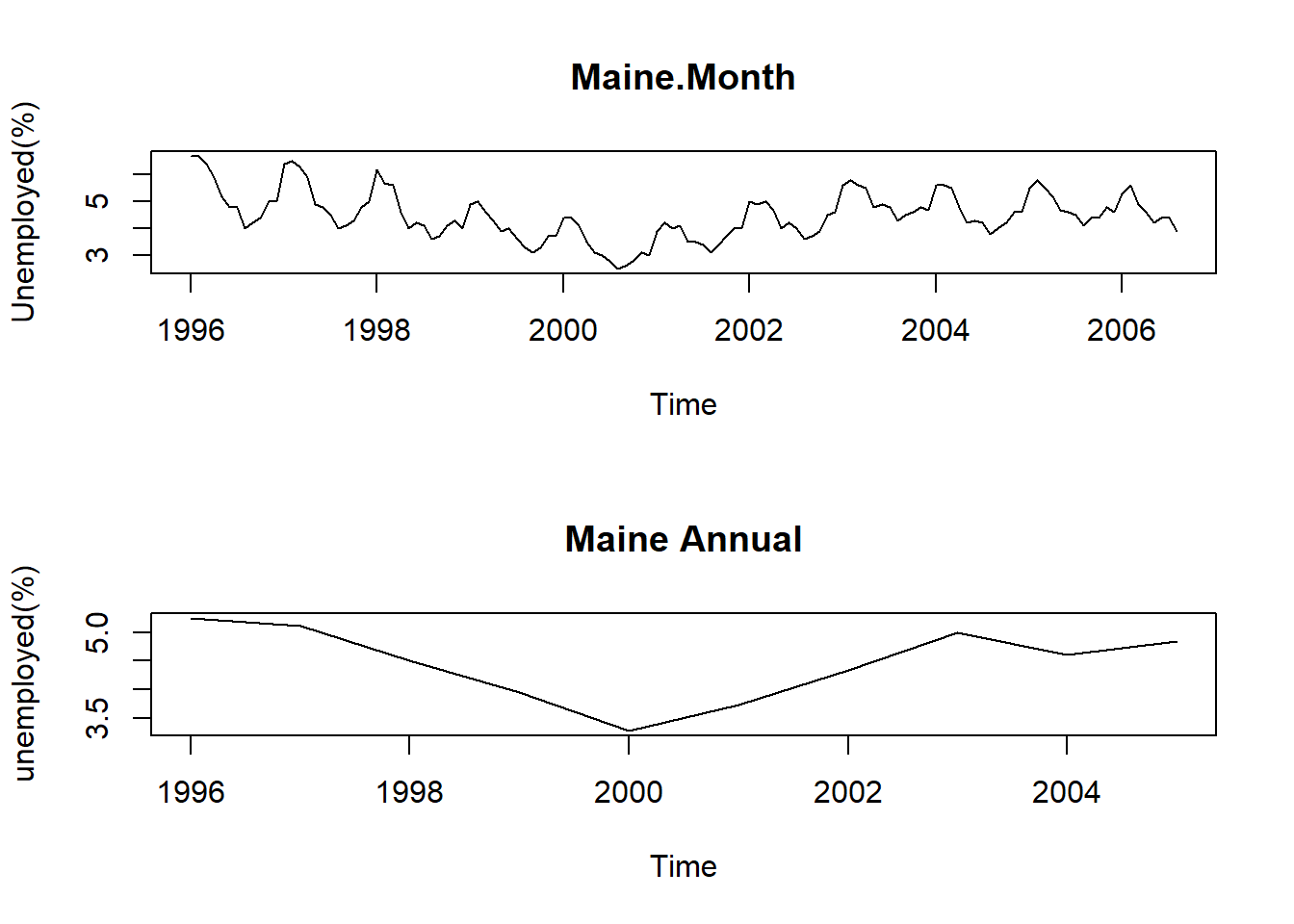### Window Function: For getting a sample of the data.

We can calculate the precise percentages in R, using window. This function will extract the part of the time series between specified start and end points. and will sample with an interval equal to frequency if its argument is set to TRUE. So, the below line below gives a time series of February figures.

Maine.Feb <- window(Maine.month.ts,start=c(1996,2),freq=TRUE)
Maine.Feb
## Time Series:
## Start = 1996.083
## End = 2006.083
## Frequency = 1
##   6.7 6.5 5.7 5.0 4.4 4.2 4.9 5.8 5.6 5.8 5.6

We can calculate similarly for August

Maine.Aug <- window(Maine.month.ts,start=c(1996,8),freq=TRUE)
Maine.Aug
## Time Series:
## Start = 1996.583
## End = 2006.583
## Frequency = 1
##   4.0 4.0 3.6 3.3 2.5 3.1 3.6 4.3 3.8 4.1 3.9

We can see that August Figures are in General Lower than Februrary. so we can calculate how much is Feb more or less as compared to the mean of all the years, similarly for August. This gives a sort of seasonal Index.

Feb.ratio <- mean(Maine.Feb)/mean(Maine.month.ts)
Aug.ratio <- mean(Maine.Aug)/mean(Maine.month.ts)
Feb.ratio
##  1.222529
Aug.ratio
##  0.8163732

So on an average unemployment in February is 22% more than the average and August is about 18% less than the average.

## US Unemployment Data

US.month <- read.table("ts/USunemp.dat",header=TRUE)
head(US.month)
##   USun
## 1  5.6
## 2  5.5
## 3  5.5
## 4  5.6
## 5  5.6
## 6  5.3

converting it into time series, plotting and comparing with the Maine unemployment rate

US.month.ts <- ts(US.month$USun, start=c(1996,1), end=c(2006,10), freq = 12) layout(1:2) plot(US.month.ts,ylab="usemployed(%)", main="US Unemployment Rate") plot(Maine.month.ts,ylab="Unemployed(%)",main="Maine Unemployment Rate")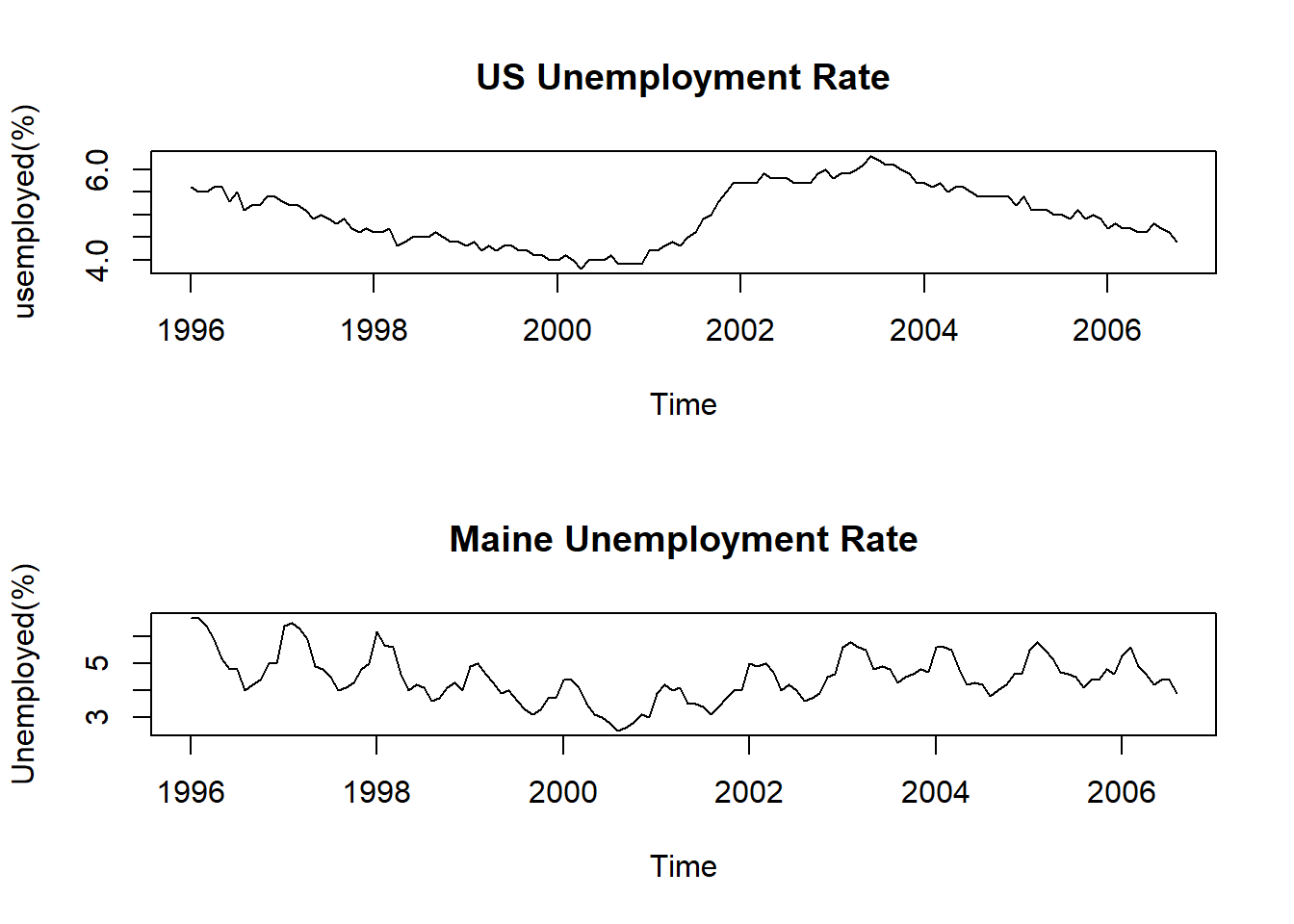## Multiple Series: Choc Beer Electricity Data Reading the data. Choc is in tonnes, beer in millions, electricity in million of Kwh. Years: Jan 1958 to Dec 1990. CBE <- read.table("ts/cbe.dat",header=TRUE) head(CBE) ## choc beer elec ## 1 1451 96.3 1497 ## 2 2037 84.4 1463 ## 3 2477 91.2 1648 ## 4 2785 81.9 1595 ## 5 2994 80.5 1777 ## 6 2681 70.4 1824 Checing the class class(CBE) ##  "data.frame" Lets create ts objects for elec, beer and choc data. Elec.ts <- ts(CBE[, 3], start = 1958, freq = 12) Beer.ts <- ts(CBE[, 2], start = 1958, freq = 12) Choc.ts <- ts(CBE[, 1], start = 1958, freq = 12) ### cbind: plot Several series in one Figure plot(cbind(Elec.ts, Beer.ts, Choc.ts), main= "Electrity ,Choc and Beer Time Series")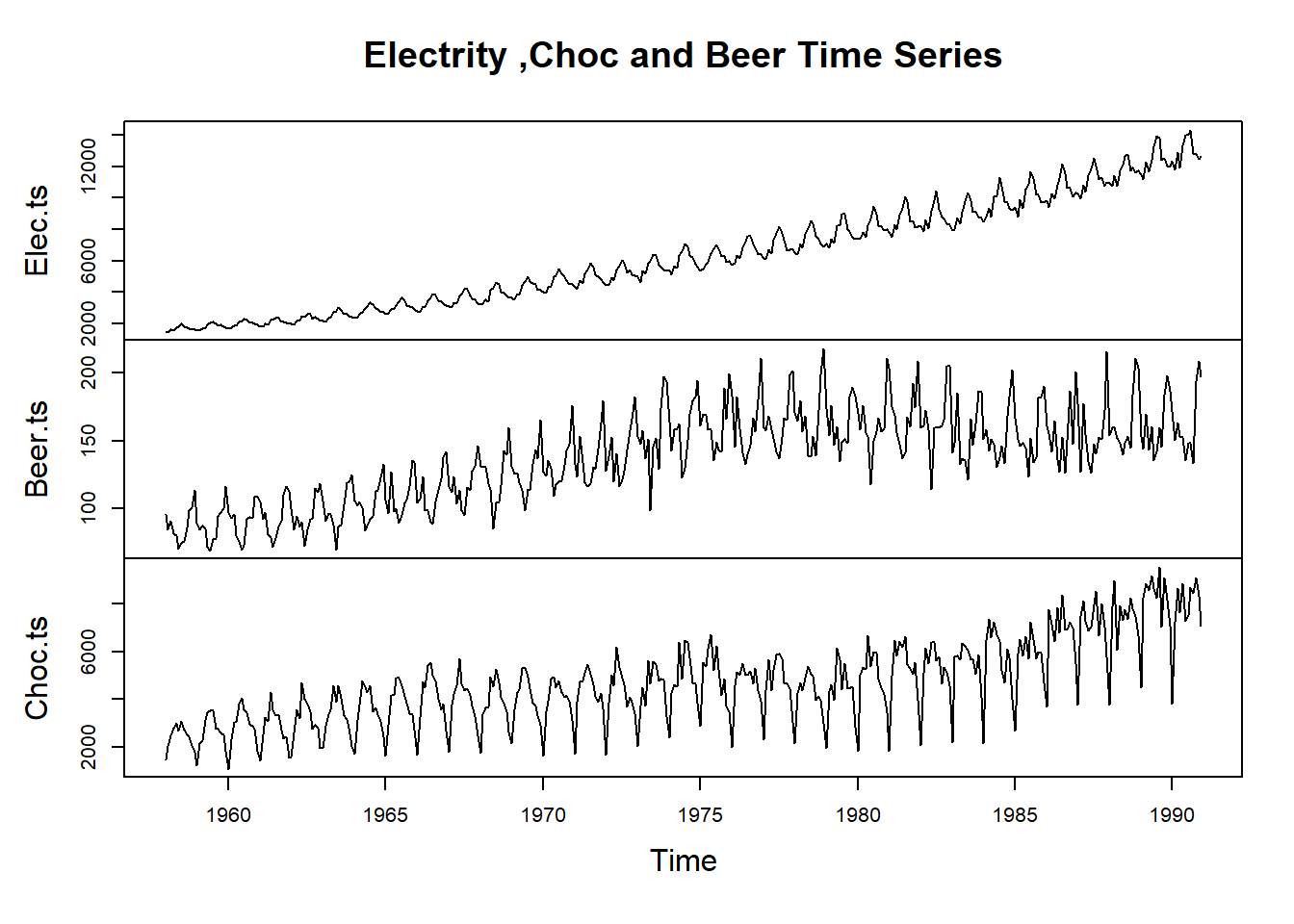### ts.intersect: To Obtain the intersection of two series Intersection between air passenger data and the electricity data AP.elec <- ts.intersect(AP,Elec.ts) Lets check the start and end of the series. Lets see a few rows of the time series start(AP.elec) ##  1958 1 end(AP.elec) ##  1960 12 AP.elec[1:3,] ## AP Elec.ts ## [1,] 340 1497 ## [2,] 318 1463 ## [3,] 362 1648 Lets extract the data for each series AP <- AP.elec[,1];Elec <- AP.elec[,2] Lets Plot these on one go layout(1:2) plot(AP, main="",ylab="Air Passengers/1000's") plot(Elec,main="",ylab="Electricity production/Mwh")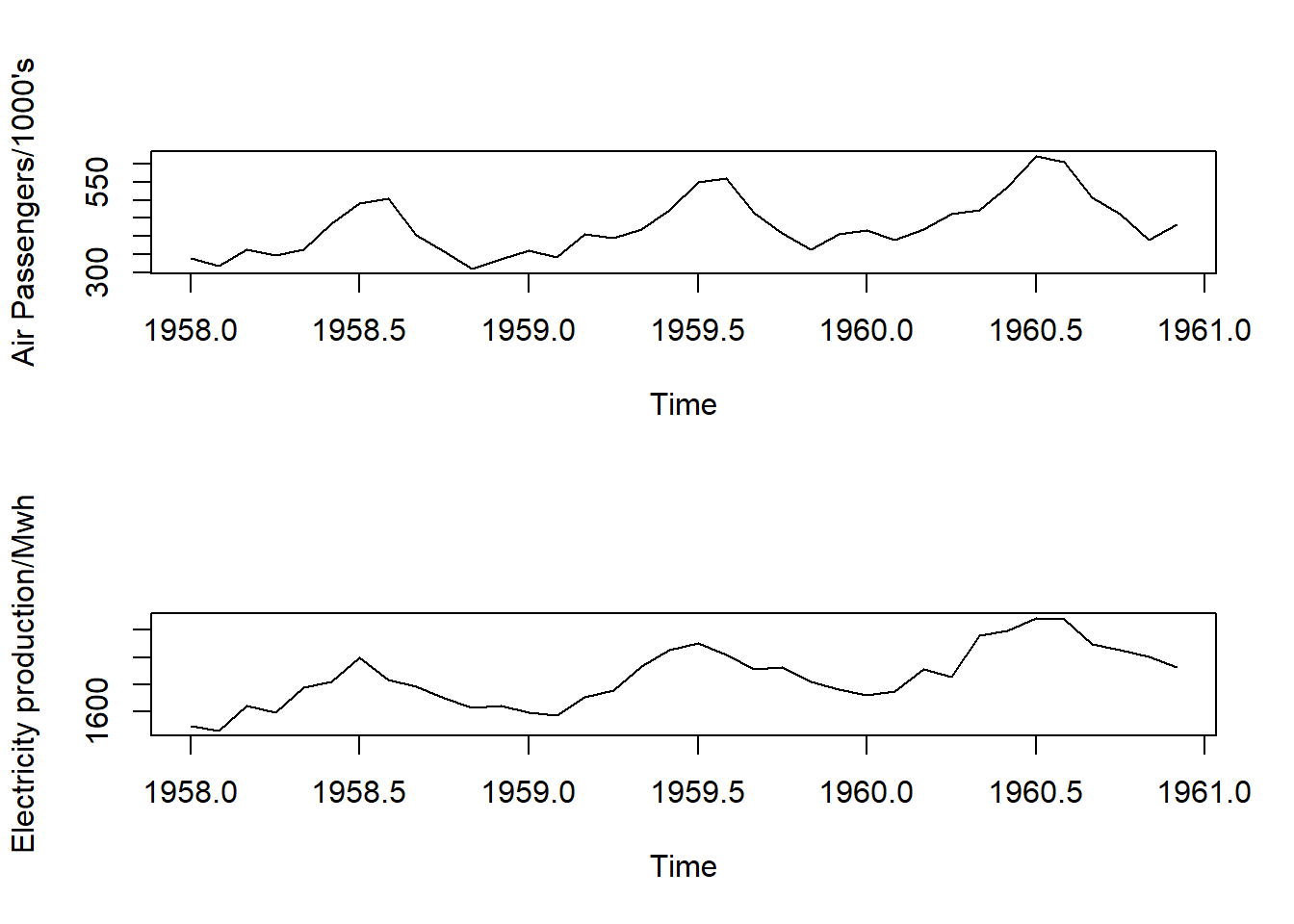Lets plot a scatter plot between the two. We need to convert the ts objects to vectors. plot(as.vector(AP),as.vector(Elec), xlab="Air passengers/1000's", ylab="Electricity production/MWh")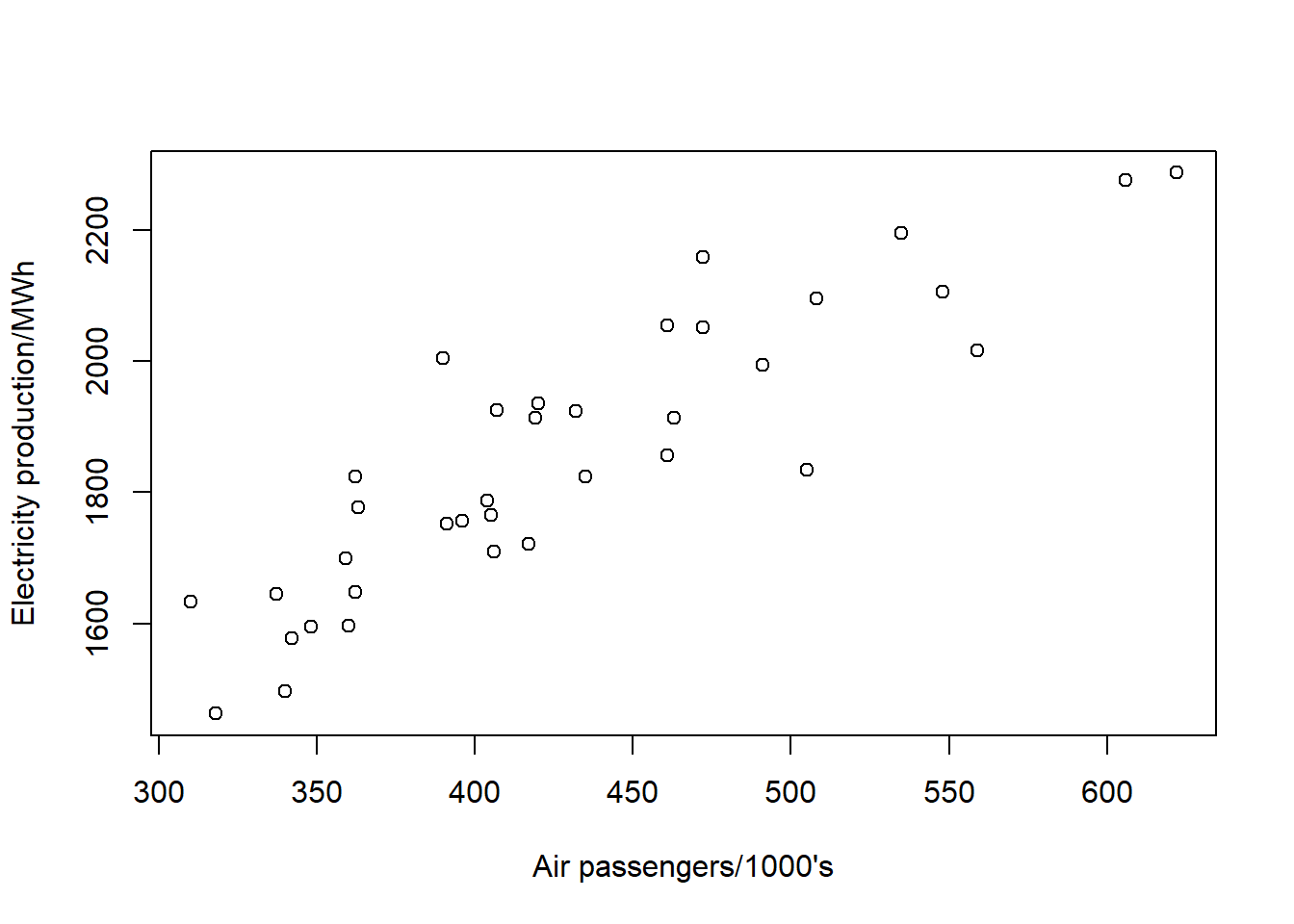Lets draw a trendline in these points plot(as.vector(AP),as.vector(Elec), xlab="Air passengers/1000's", ylab="Electricity production/MWh") abline(reg=lm(Elec~AP))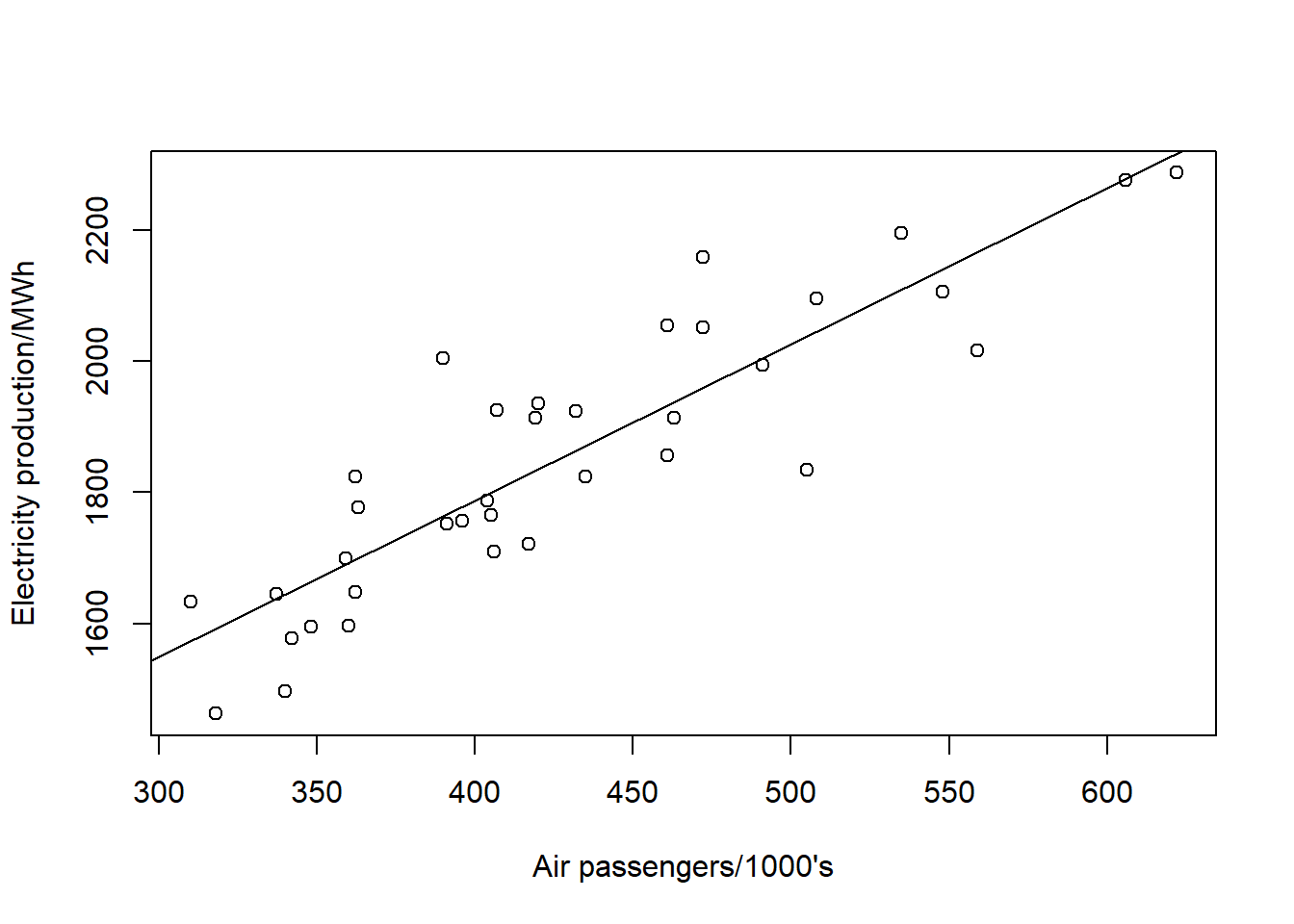This relation may be spurious. Lets have a look at correlation. cor(AP,Elec) ##  0.8841668 So the two time series are highly correlated. As told above, it could be spurious. It is not plausible that higher number of air passengers are caused by higher electricity production in Australia. ## Quarterly Exchange Rate: GBP to NZ Dollar Period: Jan 1991 to Mar 2000 Data: Mean values Frequency: Quarterly Start of Frequency: Jan- Mar End of Frequency: Oct-Dec Z <- read.table("ts/pounds_nz.dat") head(Z) ## V1 ## 1 xrate ## 2 2.9243 ## 3 2.9422 ## 4 3.1719 ## 5 3.2542 ## 6 3.3479 Lets see the summary and structure str(Z) ## 'data.frame': 40 obs. of 1 variable: ##$ V1: Factor w/ 40 levels "2.2351","2.245",..: 40 22 23 29 35 36 38 25 21 19 ...

Converting to ts object and plotting

Z.ts <- ts(Z,st=1991,fr=4)
plot(Z.ts,xlab="time/years",ylab="Quarterly Exchange Rate of $NZ/pound")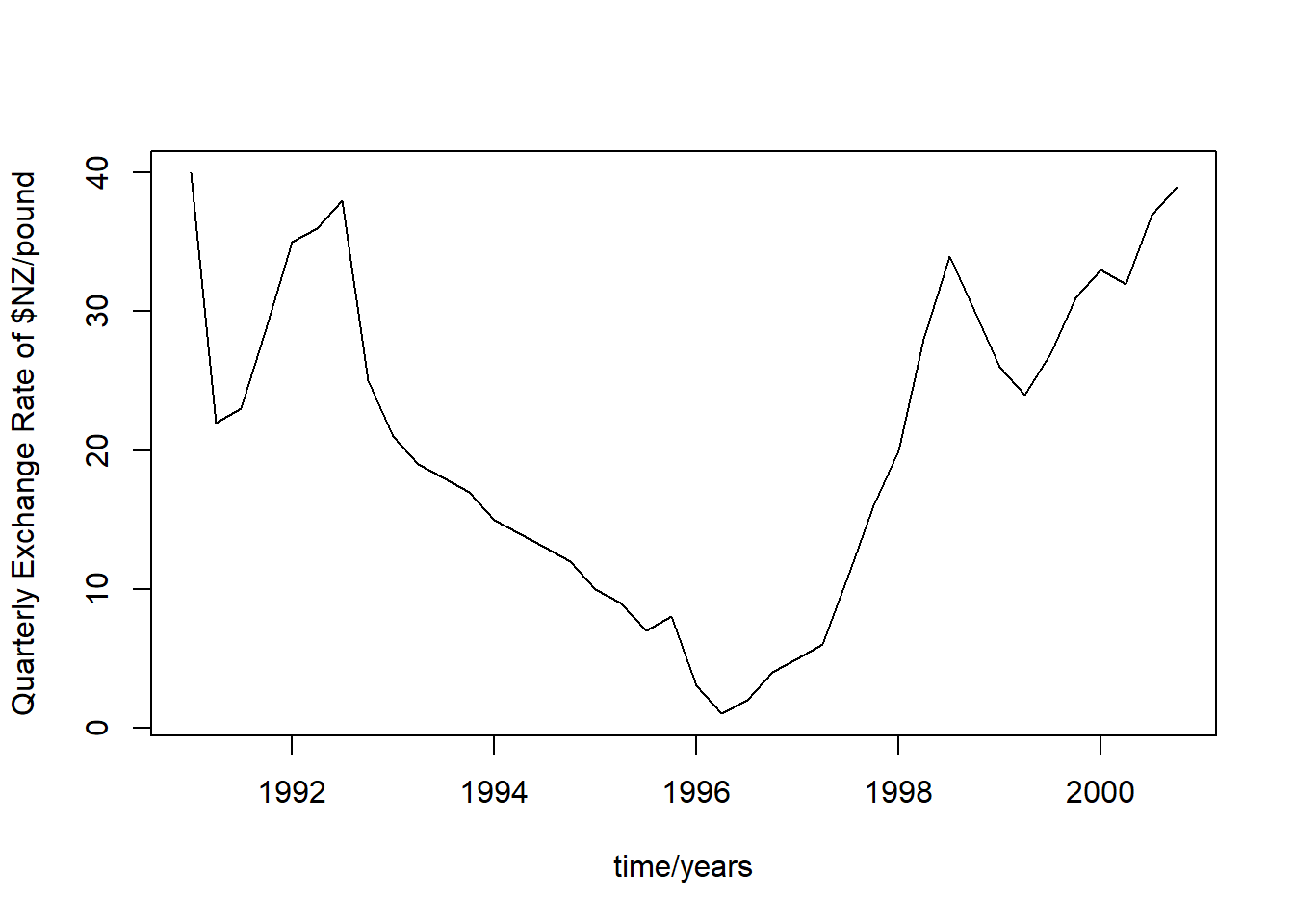We can see that there are two treends 1992-1996 and 1996-1998.The window function can be used to extract the entries. Z.92.96 <- window(Z.ts,start=c(1992,1),end=c(1996,1)) Z.96.98 <- window(Z.ts,start=c(1996,1),end=c(1998,1)) layout(1:2) plot(Z.92.96,ylab="Exchange rate in$NZ/pound",
xlab= "Time(years)")
plot(Z.96.98,ylab="Exchange rate in \$NZ/pound",
xlab= "Time(years)")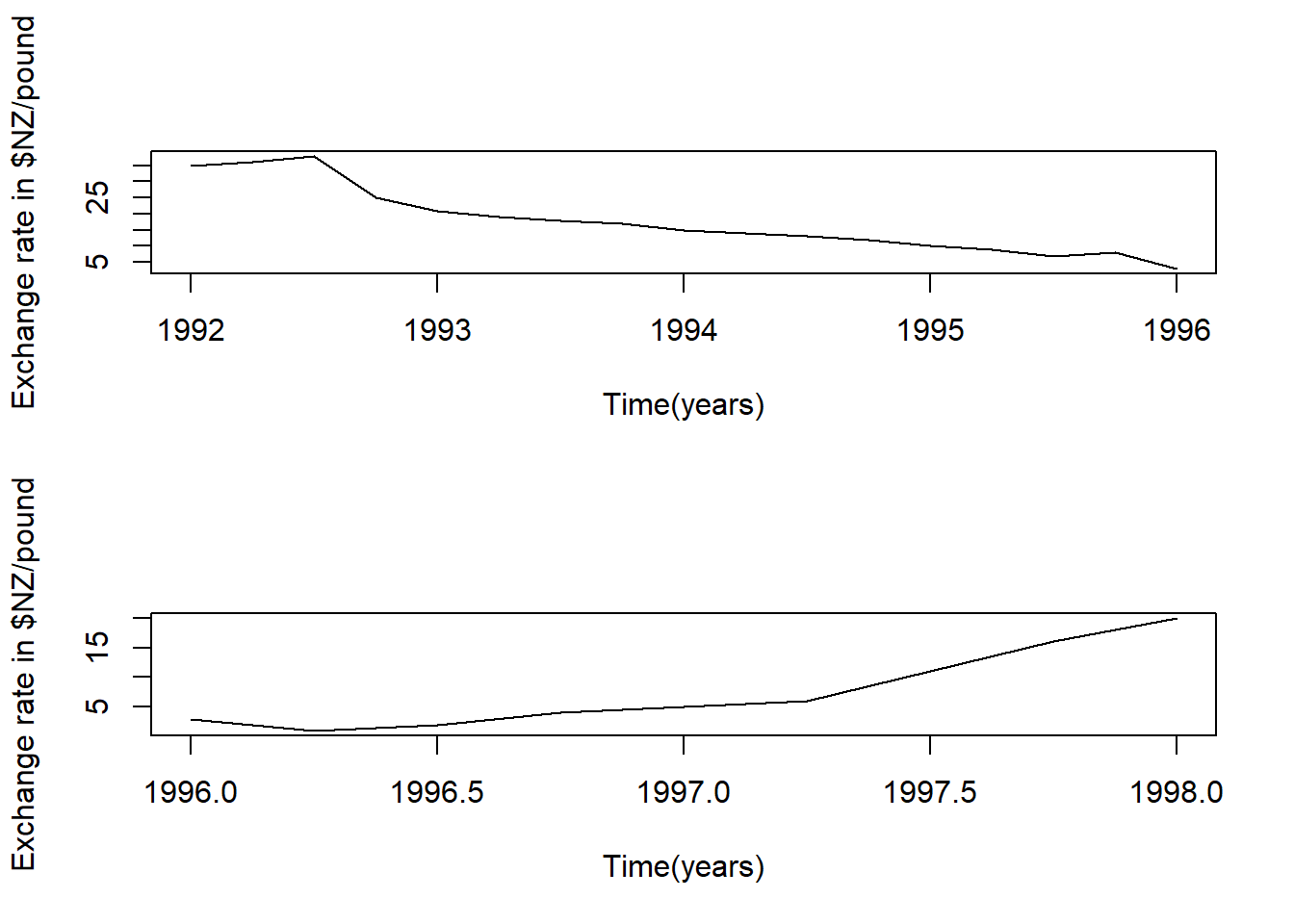If you are at the start of 92, you could see that the data is only goint down, and vice versa in the next period. This is pitfall of stochastic trend, can be reduced by statistical test for this.

## Global Temperature Dataset

Period: Jan 1856- Dec 2005 Data: Values Transformation: No Unit: In degree celcius Frequency: Monthly Start of Frequency: Jan 1856 End of Frequency: Dec 2005

### use of SCAN Function to convert a dataframe into an array. This is needed as there are moe than one columns.

Global <- scan("ts/global.dat")

Let us see the class

class(Global)
##  "numeric"

Lets convert it into a time series

Global.ts <- ts(Global, st = c(1856, 1), end = c(2005, 12),
fr = 12)

Lets plot

plot(Global.ts)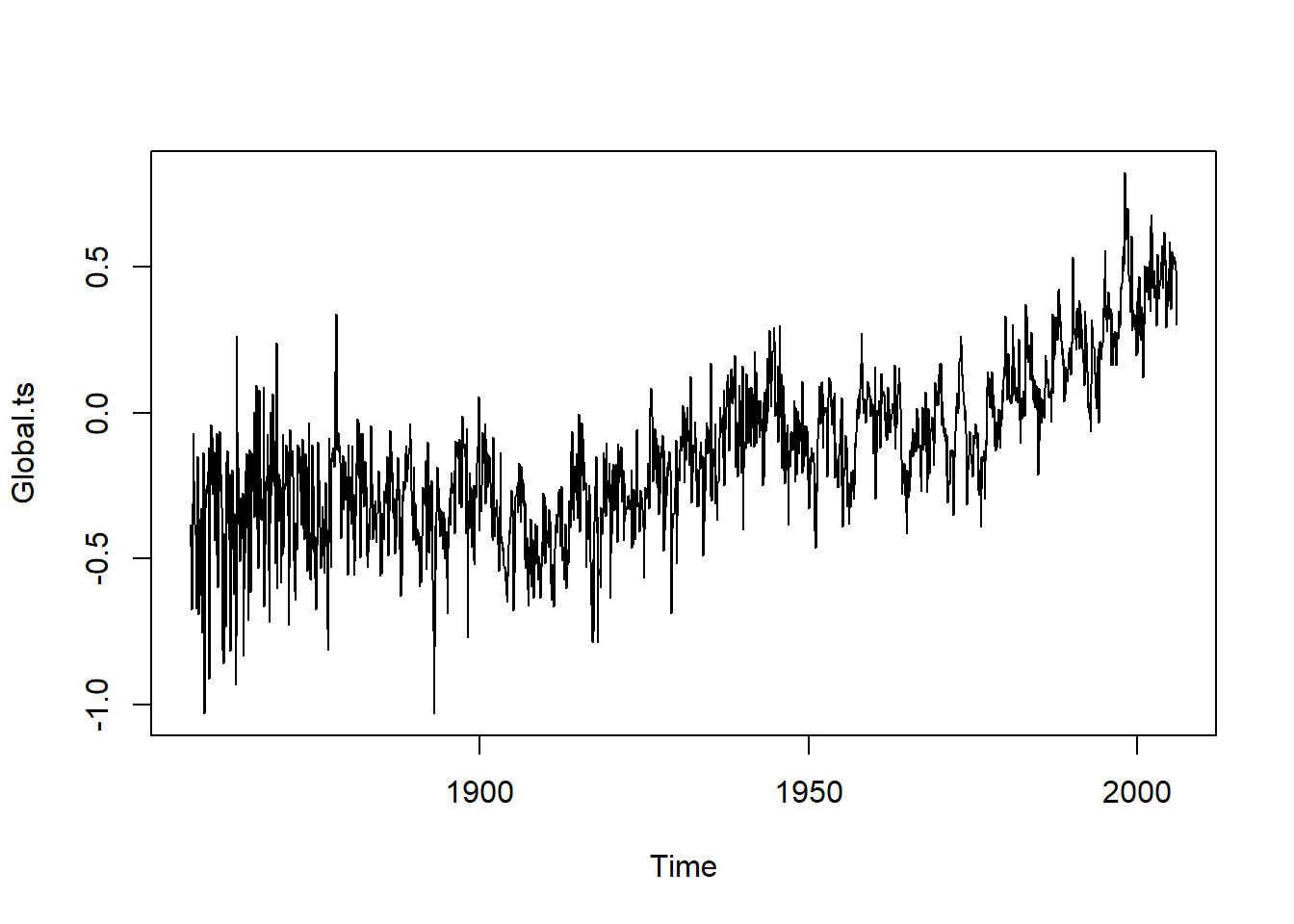Let us see the trend after removing seasonal effect.

Global.annual <- aggregate(Global.ts,FUN=mean)
plot(Global.annual)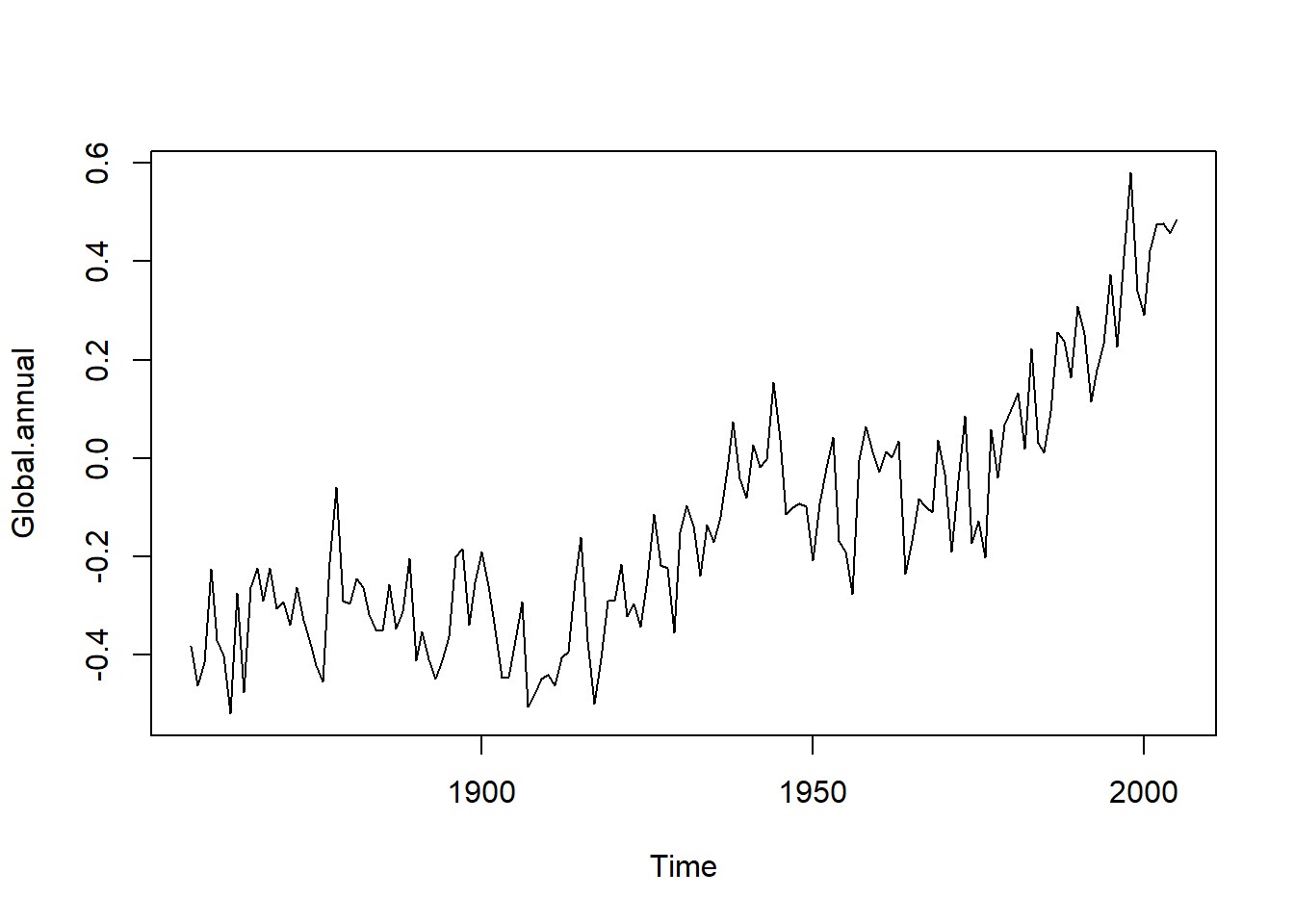The upward trend after 1970 has been used as the evidence of global warning. let us see the period from 1970 to 2005.

New.series <- window(Global.ts,start=c(1970,1),end=c(2005,12))
plot(New.series)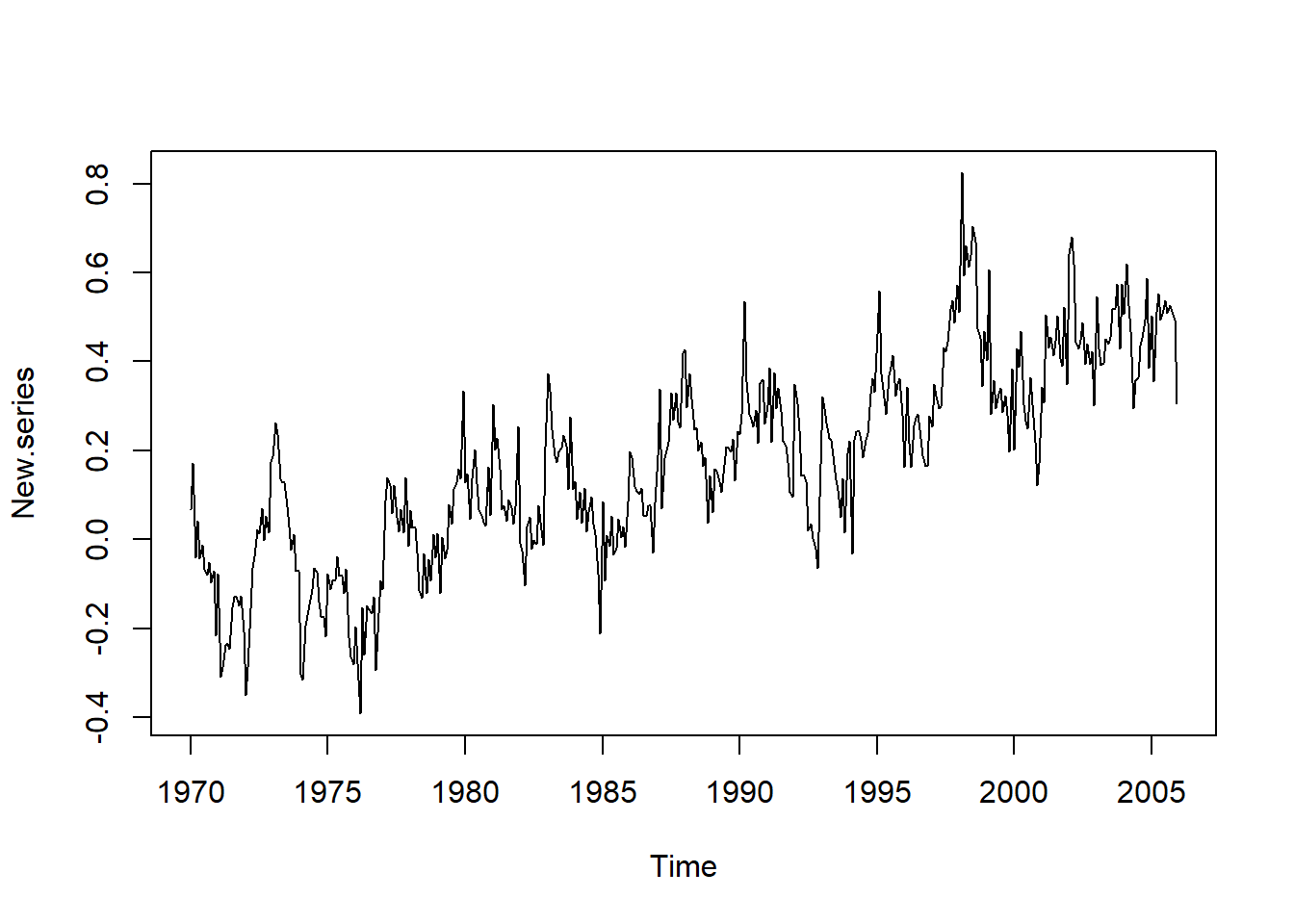### time function: To draw a regression line in the series. Lets draw a regression line in the equations.

New.time <- time(New.series)
plot(New.series)
abline(reg=lm(New.series~New.time))